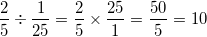## Example Questions

### Example Question #151 : Fractions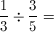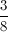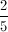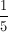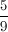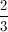Explanation:

Cross multiply or multiply using the reciprocal of the second fraction.

### Example Question #1 : How To Divide Fractions

Simplify the following expression: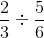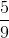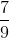Explanation: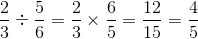### Example Question #473 : Arithmetic

Evaluate the following: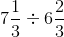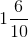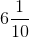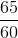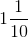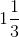Explanation:

Start by converting 71/to 22/3, and 6 2/to 20/3. We then multiply 22/3 by the reciprocal of 20/3, 3/20, and you get 66/60. This simplifies to 11/10.

### Example Question #161 : Fractions

Solve.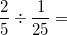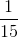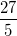Remember, to divide a number by a fraction, multiply the number by the reciprocal of the fraction. In this case,